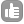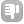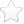0Hi all, I have a small question on excitation bandwidth for shaped pulses. In many of the literature, the field strength `( nu (Hz) = Gamma * B_1/(2 * pi))` of the shaped pulse is specified, so i use it to determine the pulse width `(t_p)` using the relations, ``````omega * t_p = theta; & Omega = 2 * pi * nu t_p= theta/(2 * pi * nu) [sec] `````` On knowing 't_p' value, i can use 'shape tools' available in Bruker to calculate the power level of the shaped pulse in comparison to a 90 degree hard pulse. My question is-- is the nu(Hz) value mentioned in the literature, the actual excitation bandwidth of the shaped pulse? Thank you! asked Jun 20 '12 at 16:56Jana 31Evgeny Fadeev 577●13Hi Jana, There are two questions here: 1) what is the meaning of the nu value quoted, and 2) how does that relate to the excitation (or inversion, or refocusing), bandwidth of the pulse?. Is there a specific example you're interested in? If you give a reference I could look at this, because the answer to 1) is not always the same: a) The field strength of the pulse that is quoted could to be [Gamma * B_1/(2 * pi)]max, i.e. the peak RF field strength. This would then correspond to the power level that you need to set in the spectrometer for that pulse. For example, if your hard 90 is 12.5us, that would be 1/(4*12.5us) = 20kHz. If the peak RF field for your shaped pulse is 5kHz, you would need 12dB more attenuation, so in Bruker units if your pl for the hard 90 is 1dB, sp for the shaped pulse would be 13dB. In watt units you would need a factor of 16 less watt (rf field is proportional to square root of power in watt)), so if your hard 90 is 16 watt your shaped pulse would be 1 watt. b) You could also specify the "nutation frequency", i.e. theta / (t_p * 2 * Pi), as per your equation above. It seems to me that it doesn't make sense to quote the nutation frequency of the pulse in this way, in general. This is because that equation may not hold for all theta or t_p values - e.g. adiabatic 180 degree pulses, where for example if you increase the pulse length, you still get a 180 degree rotation. c) Thirdly you could specify the bandwidth in Hz of the pulse. This normally means the range at which the excitation (or inversion or refocusing) drops to 70.8% of the max ( a reduction of 3dB). For example for a rectangular 90 degree pulse of 1000us, the magnitude of the transverse magnetization drops to 70.8% at +/- 558 Hz, so the bandwidth is 1116 Hz. Personally I would specify all the values independently , the flip angle, peak RF field, and bandwidth, but I don't know what the norm is. Hopefully it would be clear from the context which nu value is being used! On to question 2), how does the bandwidth relate to the nu value quoted? Obviously this depends on which nu value is used, but for a given shape envelope and flip angle there will be a fixed bandwidth factor which is equal to (bandwidth * t_p). For example, for a rectangular 90 degree pulse the bandwidth factor is 1.116, so the bandwidth is 1.116/t_p. For other shapes, and for other flip angles, this factor is different, e.g. for a 90 degree EBURP-2 pulse, which gives a very "top-hat" excitation profile, the bandwidth factor is 4.952. Bandwidth factors for a variety of pulses are listed in e.g. Tim Claridge's book. They are also stored in the files describing the shape. To summarise: You need to know two things: The definition of nu being used, and the bandwidth factor. If you know the pulse length, then it's clear which definition is being used, because you can check against the equations nu = theta / (t_p * 2 * Pi) and (bandwidth factor) = bandwidth * t_p. You can then calculate the bandwidth if required, or pulse length. Finally to calculate the peak RF field strength required, you need to know the integral ratio compared to a rectangular pulse. For example, a gaussian pulse has an integral of 41.2% of a rectangle. This means you need the peak RF field to be 2.43 * larger than a rectangular pulse of the same length, corresponding to 7.71dB less attenuation. In shape tool, the menu options Analysis-> Calculate bandwidth for excitation/inversion/refocusing allows you to calculate the length required for a given bandwidth. Once you have this length, you can use Analysis-> Integrate shape to calculate the required power level, in terms of the difference from the hard pulse power. Hope this helps! answered Jul 01 '12 at 15:44Pete Gierth 401 Thanks Pete Gierth, for clearly detailing all the different aspects of the shape pulse calculations. - Jana (Jul 02 '12 at 11:43)1Hi, There isn't as such a bandwidth factor for HypSec pulses in general, because they are adiabatic, the bandwidth depends on the frequency sweep used. In the original paper by Hoult they notate the pulse in terms of parameters beta and mu, where the amplitude profile is given by B1(t) = B1(0) sech (beta*t) and the frequency sweep profile by: Omega(t) = - (mu * beta) tanh (beta * t) and t goes from -Tp/2 to +Tp/2 (Tp = pulse length) for adiabatic full passage pulses as used for inversion. One complication is that we typically create shaped RF pulses as digitised amplitude and phase lists - the frequency modulation is implemented as a phase modulation. Thus if we change the pulse length, the rate of phase modulation changes and therefore the total frequency modulation changes, as does the effective beta value. So if we create a given shape file with specified mu value, that is based on a particular assumption about the pulse length. I don't know how this is handled in imaging software, but in Topspin HypSec pulses are created with their numbers assuming a 1 second pulse length. The "true" beta and mu values will depend on the actual length of the pulse as applied (i.e. how long each digital step of the shape lasts for). In topspin one can also define the pulse in terms of truncation level and frequency sweep range (relative to a 1s pulse). If you want to use for example a 10ms pulse, then the sweep range you set needs to be 0.01 * the desired sweep range. The bandwidth is close to Omega(-Tp/2) - Omega(Tp/2) (=the sweep range) which you can calculate from the mu and beta values if the pulse is specified in that way. In fact the frequencies Omega(-Tp/2) and Omega(-Tp/2) define the points at which the magnetization will be rotated through 90 degrees. The sharpness of the inversion profile depends on the mu value based on a fixed length pulse - increasing mu means a sharper profile, although at a cost of increased pulse length for a given bandwidth. Thinking in terms of setting truncation level and sweep range, a sharper inversion profile is achieved by setting a larger sweep range and making the pulse longer. However, for a given defined shape file, scaling the length of the pulse inversely scales the bandwidth. That is, if we make the pulse take twice as long, the bandwidth is halved. So for a given shape defined by particular mu and beta values (relative to a 1 second pulse), we could define a bandwidth factor. But I don't know if we can write a simple expression for the bandwidth factor based on mu and beta... Does this help you at all? Pete answered Sep 30 '13 at 14:35Pete Gierth 4010Hello Pete Gierth, i tried to find the bandwidth factor for an adiabatic pulse (hyperbolic secant) everywhere fruitless. Do you know the bandwidth factor and can tell me or give me a hint where I can find it? I need it for my Bachelor thesis. I dont want to buy the book of Timothy Claridge. Thanks so much. answered Sep 24 '13 at 14:31